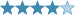## Cyclometer

(Eingestellt am 19. September 2021, 03:38 Uhr von SirSchmoopy)

Special thanks to Sktx for testing this.

I believe the examples given below should clarify things pretty well, but let me know if you still have trouble understanding the rules or have any other feedback.Standard Rules:
• Normal Sudoku Rules Apply
• Normal Thermo Rules Apply. Along thermometers, digits increase starting from the bulb
• Normal Killer rules apply. Digits in cages sum to the amount indicated in the top left corner.

Definitions:
• Let a cycle be defined as the sequence of digits starting at digit A in column X, then digit B in column A, then digit C in column B, then digit D in column C....
(for this puzzle, only horizontal cycles/ traversing across columns are considered)
• Let the order of a cycle be defined as the number of unique digits contained in a cycle. (so if a cycle is 9 -> 1 -> 9, it would have an order of 2)
Cycle Rules:
• Along thermometers, the order of the cycles strictly increases starting from the bulb
• In killer cages, the sum of the orders of the cycles is equal to the total indicated in the top left corner. Orders are allowed to repeat within a cage (so a 6 cage could have 2 order 3 cycles in it for example)
Examples:

See the below for some examples which are valid using the above rules. I've also illustrated the cycles (the color is just to disambiguate and isn't significant)

For the killer cage:

• The order of the 4 in the cage is 3 (4 -> 5 -> 8)
• The order of the 2 in the cage is 3 (2 ->3 -> 9)
• 4 + 2 = 3 + 3 = 6
• as above, orders can repeat within cages

For the thermo:

• The 1's cycle has an order of 1, and the cycle is just the digit 1
• The 3's cycle has an order of 2, and the cycle is 3 -> 1
• The 6's cycle has an order of 4, and the cycle is 6 -> 5 -> 4 -> 1
• 1 < 3 < 6 and 1 < 2 < 4 (note these are all strict inequalities)

•Lösungscode: Digits in row 1 and 8, left to right without spaces (e.g. 123456789234567891)

Zuletzt geändert am 19. September 2021, 05:57 Uhr

Gelöst von Arashdeep Singh, grkles
Komplette Liste

### Kommentare

am 22. September 2021, 17:01 Uhr von grkles
my goodness, that was so hard! quite rewarding, though.

am 20. September 2021, 05:37 Uhr von Arashdeep Singh
Very difficult and very nice logic. Great stuff.

am 19. September 2021, 05:57 Uhr von SirSchmoopy
clarified rule that cycles are strictly horizontal/travelling across columns

 Schwierigkeit:Bewertung: N/A Gelöst: 2 mal Beobachtet: 0 mal ID: 0007NF

Lösungscode:

## Anmelden